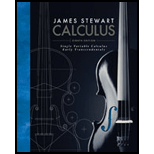Chapter 10.5, Problem 43E### Single Variable Calculus: Early Tr...

8th Edition
James Stewart
ISBN: 9781305270336

#### Solutions

Chapter
Section### Single Variable Calculus: Early Tr...

8th Edition
James Stewart
ISBN: 9781305270336
Textbook Problem

# Find an equation for the conic that satisfies the given conditions.43. Hyperbola, vertices (±3, 0), foci (±5, 0)

To determine

To Find: The equation for the conic using the vertices (±3,0) and foci (±5,0) of the Hyperbola.

Explanation

Given:

The vertices and foci of the hyperbola are (±3,0) and foci (±5,0).

Calculation:

The foci and vertices are located in x axis.

Therefore, the center of the hyperbola (h,k) is (0,0).

Find the value of a the equation of foci as below.

vertices=((h±a),k)

Substitute (0,0) for (h,k) and (±3,0) for vertices.

(±3,0)=((h±a),k)(0±a)=±3a=±3

The value of a is +3.

Compute the value of c using the equation below.

foci=(h,(k±c))

Substitute (0,0) for (h,k) and (±5,0) for foci.

(±5,0)=((0±c),0)±5=(0±c)c=±5

Therefore, the value of c is ±5

### Still sussing out bartleby?

Check out a sample textbook solution.

See a sample solution

#### The Solution to Your Study Problems

Bartleby provides explanations to thousands of textbook problems written by our experts, many with advanced degrees!

Get Started

## Additional Math Solutions

#### Find more solutions based on key concepts